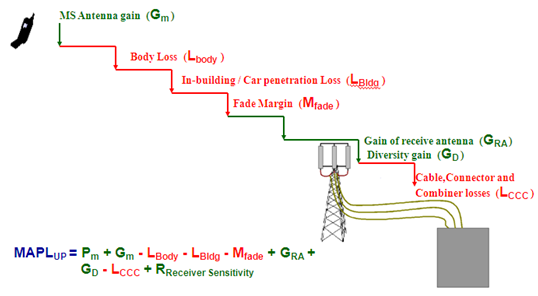Receiver sensitivity is the ability of the receiver to receive signals in the sense that any signal below the sensitivity is considered as noise and is not usable.

S = Antenna Noise (dBm) + Receiver Noise Figure (dB) + C/N (dB)

C/N = Carrier to noise ration required in the presence to achieve a specified BER.

Antenna Noise (dBm) = 10log (kTB)

Where k = Boltzmann constant 1.38 X 10-20 milli Joules / Kelvin

T = Room temperature in degrees kelvin

B = Bandwidth in HzIn A Nutshell

• Mobile Transmit power
• Mobile antenna gain
• Body Loss